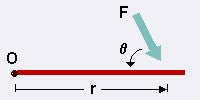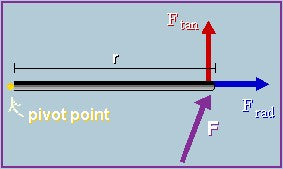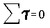Torque is a measure of how much a force acting on an object causes that object to rotate. The object rotates about an axis, which we will call the pivot point, and will label 'O'. We will call the force 'F'. The distance from the pivot point to the point where the force acts is called the moment arm, and is denoted by 'r'. Note that this distance, 'r', is also a vector, and points from the axis of rotation to the point where the force acts. (Refer to Figure 1 for a pictorial representation of these definitions.)Figure 1 Definitions Torque is defined as       𝜏 = r x F = r F sin(θ). In other words, torque is the cross product between the distance vector (the distance from the pivot point to the point where force is applied) and the force vector, 'a' being the angle between r and F. Using the right hand rule, we can find the direction of the torque vector. If we put our fingers in the direction of r, and curl them to the direction of F, then the thumb points in the direction of the torque vector. Imagine pushing a door to open it. The force of your push (F) causes the door to rotate about its hinges (the pivot point, O). How hard you need to push depends on the distance you are from the hinges (r) (and several other things, but let's ignore them now). The closer you are to the hinges (i.e. the smaller r is), the harder it is to push. This is what happens when you try to push open a door on the wrong side. The torque you created on the door is smaller than it would have been had you pushed the correct side (away from its hinges). Note that the force applied, F, and the moment arm, r, are independent of the object. Furthermore, a force applied at the pivot point will cause no torque since the moment arm would be zero (r = 0).
 Another way of expressing the above equation is that torque is the product of the magnitude of the force and the perpendicular distance from the force to the axis of rotation (i.e. the pivot point).Figure 2 Tangential and radial components of force F Let the force acting on an object be broken up into its tangential (Ftan) and radial (Frad) components (see Figure 2). (Note that the tangential component is perpendicular to the moment arm, while the radial component is parallel to the moment arm.) The radial component of the force has no contribution to the torque because it passes through the pivot point. So, it is only the tangential component of the force which affects torque (since it is perpendicular to the line between the point of action of the force and the pivot point). There may be more than one force acting on an object, and each of these forces may act on different point on the object. Then, each force will cause a torque. The net torque is the sum of the individual torques. Rotational Equilibrium is analogous to translational equilibrium, where the sum of the forces are equal to zero. In rotational equilibrium, the sum of the torques is equal to zero. In other words, there is no net torque on the object.Note that the SI units of torque is a Newton-meter, which is also a way of expressing a Joule (the unit for energy). However, torque is not energy. So, to avoid confusion, we will use the units N.m, and not J. The distinction arises because energy is a scalar quantity, whereas torque is a vector.